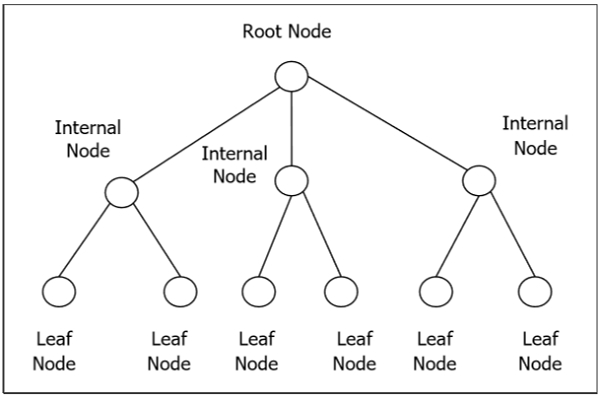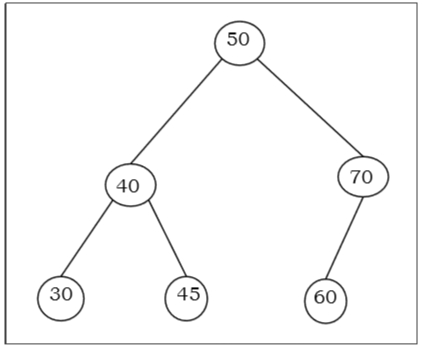# Rooted and Binary Tree

## Rooted Tree

A rooted tree G is a connected acyclic graph with a special node that is called the root of the tree and every edge directly or indirectly originates from the root. An ordered rooted tree is a rooted tree where the children of each internal vertex are ordered. If every internal vertex of a rooted tree has not more than m children, it is called an m-ary tree. If every internal vertex of a rooted tree has exactly m children, it is called a full m-ary tree. If m = 2, the rooted tree is called a binary tree.## Binary Search Tree

The binary Search tree is a binary tree which satisfies the following property −

• X in left sub-tree of vertex V, Value(X) ≤ Value (V)
• Y in right sub-tree of vertex V, Value(Y) ≥ Value (V)

So, the value of all the vertices of the left sub-tree of an internal node V are less than or equal to V and the value of all the vertices of the right sub-tree of the internal node V are greater than or equal to V. The number of links from the root node to the deepest node is the height of the Binary Search Tree.

## Example## Algorithm to search for a key in BST

BST_Search(x, k)
if ( x = NIL or k = Value[x] )
return x;
if ( k < Value[x])
return BST_Search (left[x], k);
else
return BST_Search (right[x], k)

## Complexity of Binary search tree

Average CaseWorst case
Space ComplexityO(n)O(n)
Search ComplexityO(log n)O(n)
Insertion ComplexityO(log n)O(n)
Deletion ComplexityO(log n)O(n)